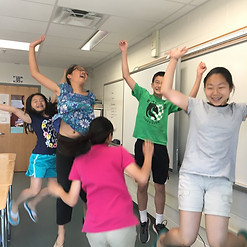top of page

The course is designed to run in parallel with regular in-school math instruction. The class will both focus on reinforcing the objectives of the students’ in-school classes, as well as introducing topics and training students to think in ways that may not be covered in the public school curriculum. Students will explore practical examples and application of mathematics and use effective learning strategies to break down new topics into constituent parts in order to better understand it.

Our teachers accomplish this by explaining complex topics in concrete terms. Only after students have acquired an understanding in a concrete context do teachers move on to abstract understanding of material. This helps students both as a means of understanding the material as well as a means of understanding the relevancy of the material. Our teachers aim to promote an intrinsic understanding of the value of math and instill in students a desire to learn – something that will help them throughout their academic lives.

For 3rd grade level, students will focus on the following priorities:

• Name and write fractions and mixed numbers represented by a model

• Create and solve single-step practical problems that involve multiplication and division through 10 x 10

• Investigate and describe the concept of probability as a measurement of chance and list possible outcomes for a single event

• Create equations to represent equivalent mathematical relationships

For 4th grade level, students will focus on the following priorities:

• Compare and order fractions and mixed numbers, with and without models

• Create and solve single-step and multistep practical problems involving addition, subtraction, and multiplication, and single-step practical problems involving division with whole numbers

• Determine the likelihood of an outcome of a simple event and represent probability as a number between 0 and 1

• Recognize and demonstrate the meaning of equality in an equation

For 5th grade level, students will focus on the following priorities:

• Create and solve single-step and multistep practical problems involving addition, subtraction, and multiplication of decimals, and create and solve single-step practical problems involving division of decimals

• Differentiate among perimeter, area, and volume and identify whether the application of the concept of perimeter, area, or volume is appropriate for a given situation

• The student will identify, describe, create, express, and extend number patterns found in objects, pictures, numbers and tables.

• Represent, interpret, and compare data represented in line plots and stem-and-leaf plots

For 6th grade level, students will focus IAAT test prep.

For middle school students, we offer

• TJ Prep

• Algebra 1

• Algebra 2

• Pre-Calculus: Precalculus will cover the skills necessary to be successful in future calculus studies. The following topics will be covered: Number Systems Review, Linear Functions and Equations, Linear Inequalities, Quadratic Functions, Polynomial and Rational Expressions, Exponentials and Logarithms,Trigonometric Functions, Trigonometry, Graphing Functions and Sequences and Series.

• AP-Calculus AB: This class will cover the topics covered in the AP Calculus course. The full AP Calculus course covers: Limits of Functions, The Derivative, Applications of Derivative, Integrals, Applications of Definite Integrals, Logarithmic and Exponential Functions and Inverse Trigonometric Functions. With a normal AP Calculus course taking 32 weeks, it is expected at least half the course will be covered in the time allotted, more depending on student pacing and prior knowledge. Interactive slides and powerpoints will be utilized along with supplemental problems from the Barron's AP Calculus Premium.